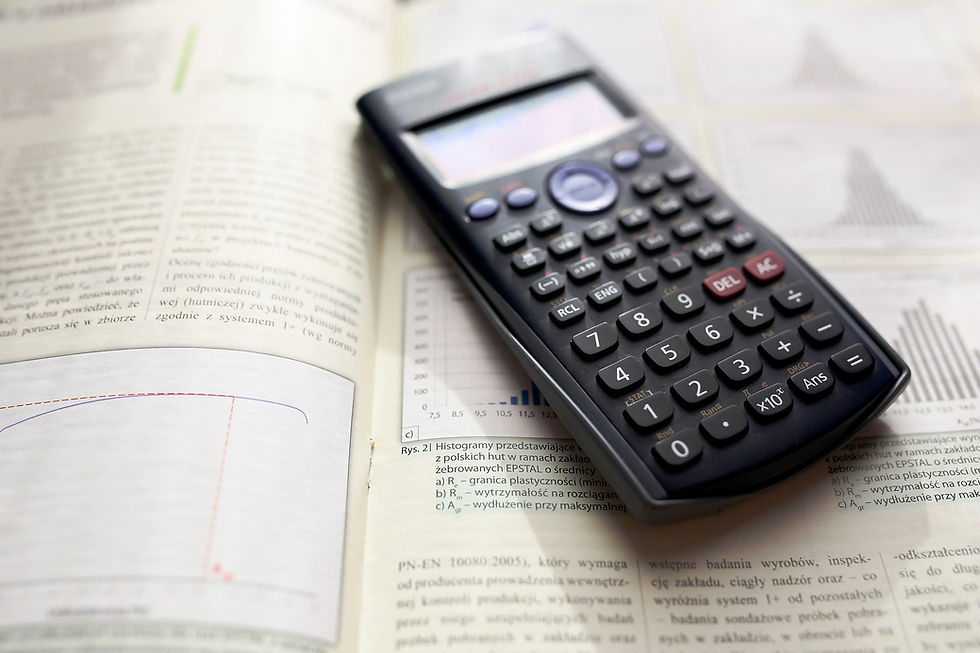top of page

# A Primer on the Brier Skill ScoreThe Brier Skill Score (BSS) is a metric that I find very useful for evaluating the performance of a dichotomous classification model (a model that is attempting to predict one of two possible outcomes). I used the BSS in my evaluation of the Kalshi prediction markets and it is my go-to evaluation metric, particularly when there is a class imbalance in the outcomes that I’m trying to predict. If you are trying to build a model to predict some rare event like a security breech or a rare disease, a standard metric like area under the receiver operating characteristic curve (AUROC) will provide little value because it is going to be heavily influenced by correct predictions of non-events (the security breech not occurring, or the absence of the rare disease). But any bad model should predict these things well since they happen most of the time. In these instances, we need a way to evaluate how good the model is at predicting the rare event, and that is when I turn to the BSS.

The BSS incorporates two main characteristics of a model’s predictive ability into a single score: calibration and confidence.

Calibration refers to the accuracy of the probability that the model assigns for each prediction. When a well-calibrated model gives us a probability of 0.70 for the outcome, the outcome should happen 70% of the time. When a poorly calibrated model gives us a probability of 0.70, the outcome may end up happening 90% of the time, or maybe only 40%, but not 70%, and that is a problem, because that means that we can’t trust what the model is telling us.

Confidence refers to how frequently our model makes well-calibrated predictions that are far away from the base event rate. If we are trying to predict something that happens 5% of the time and our model just outputs a probability of 0.05 for every prediction, it is a perfectly calibrated model, and it is also useless. We need it to be much more confident, giving us probabilities as close to 1 as possible on the occasions that the outcome actually did occur, and as close to 0 as possible on the occasions that it didn’t. The BSS quantifies how well a model does this.

THE MATH

To calculate the BSS, we first calculate two Brier Scores. What is a Brier Score? Great question! A Brier score is the sum of the squared differences between the model probability and the outcome divided by the total number of observations….What? Was that just a bunch of random words strung together in a sentence? Sorry...let me try to make that more meaningful. We need a data set that has a bunch of probabilities that the model gave for the outcome as well as whether the outcome actually happened or not. We code the outcome ‘1’ if the event occurred and ‘0’ if it did not:

Probability

Outcome

.85

1

.07

0

.35

0

.22

0

.37

0

.67

1

.18

0

.04

0

.58

0

.29

0

In the above example, the outcome occurred twice out of 10 observations, so it has a base rate of 20%. The first thing we’ll do with this is calculate a reference Brier Score, which is the squared difference between the outcome and the base rate, summed and divided by the total number of observations (in this case, 10):

 Base Rate Outcome (Outcome - 0.20) squared .20 1 0.64 .20 0 0.04 .20 0 0.04 .20 0 0.04 .20 0 0.04 .20 1 0.64 .20 0 0.04 .20 0 0.04 .20 0 0.04 .20 0 0.04 ​ Reference Brier Score 0.16

The Brier Score should always be a value between 0 and 1, with scores closer to zero being better. The base rate Brier Score is not bad. Let’s do the same thing as above, but use the model’s probability instead of the base rate.

 Probability Outcome (Outcome - Prob) squared .85 1 0.0225 .07 0 0.0049 .35 0 0.1225 .22 0 0.0484 .37 0 0.1369 .67 1 0.1089 .18 0 0.0324 .04 0 0.0016 .58 0 0.3364 .29 0 0.0841 ​ Model Brier Score 0.08986

The model Brier Score is closer to zero, so it is better than the reference Brier (as we would hope…otherwise, our model is not useful at all).

Now, to calculate the Brier Skill Score, we take the ratio of the model Brier and the reference Brier and subtract it from 1:A BSS of 1 is the best score possible. The better the model is, the closer the model Brier Score will be to zero, and the closer the BSS will be to 1. If the model and the reference Brier Scores are the same, you will get a BSS of 0 (meaning the model is no better than just predicting the base rate), and if the model is worse than predicting the base rate, the BSS will be negative (a sure sign that you have more model building work to do).

If you play around with the numbers above, you’ll see that you can get the model Brier Score to do better and better if the probabilities get closer to the outcome scores (confidence), and this will end up improving the BSS.

I realize that I got deep into the details in this post. Hopefully that is what you were looking for. If you found this useful, please share and if you have a project that you would like to pursue, please contact us at info@cwdatasolutions.com.